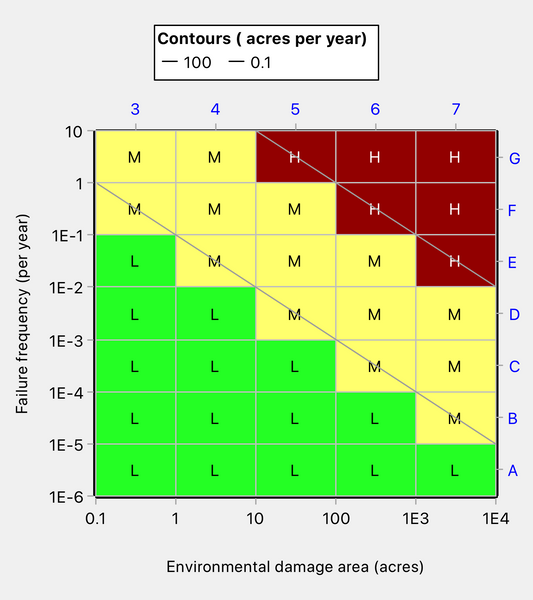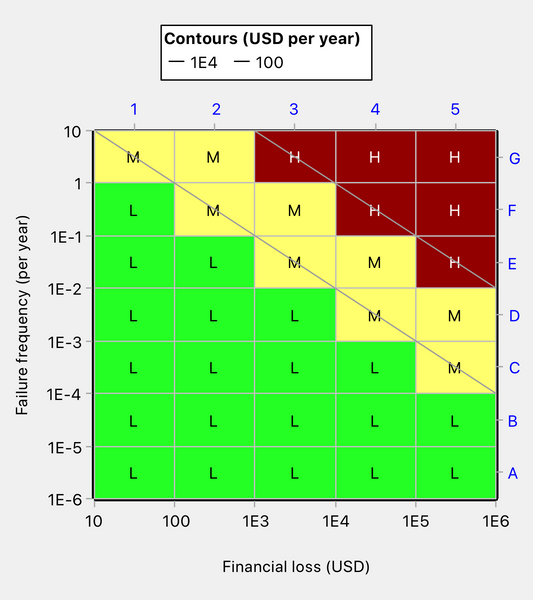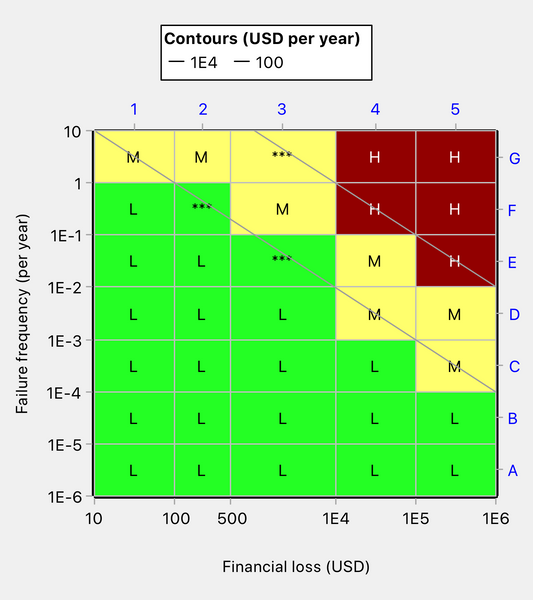# When Can the Same Risk Matrix be Used with Different Axis Scales?

The visual elements of any well-designed risk matrix are:

• A ratio scale for its probability (or frequency) axis
• A ratio scale for its consequence axis
• Contours of equal risk (iso-risk contours), which define the thresholds between the different risk priority levels
• A set of colors to visually differentiate the different risk priority levels

In addition, an algorithm is needed for deciding the color of cells that are intersected by one or more iso-risk contours. These cells, which we call “split cells” for brevity, are not located in a single risk priority level. Different parts of a split cell lie in different risk priority levels. Each split cell must be allocated to just one of the risk priority levels that it straddles. Quick Risk Matrix offers a choice of several algorithms by which to determine the coloring of the split cells.

Now, many organizations like to use a single risk matrix coloring pattern but in conjunction with different axis scales. Let’s invent an example.

Suppose an organization develops a risk matrix for the consequence type of environmental damage as shown in Figure 1 and wishes to apply the same matrix coloring scheme to the consequence type of financial loss. What are the circumstances in which it may do so?Figure 1: Risk matrix for environmental damage

In switching the horizontal axis from environmental damage to financial loss, there will be no impact on the matrix coloring scheme if the tick mark values on the horizontal axis are multiplied by a factor and if the iso-risk contours, which define the risk priority levels, are multiplied by the same factor. Let’s say that the factor will be 100. Then the risk matrix for financial loss is as shown in Figure 2, and it has the same coloring pattern as the one for environmental damage shown in Figure 1.Figure 2: Risk matrix for financial loss

So far, so good. Our fictitious organization can have a standard risk matrix coloring as shown in Figure 1 or 2 and use it in relation to both environmental damage and financial loss.

But what if the organization feels that an expected annual financial loss of \$10 is trivially low? Perhaps it would be willing to categorize risks as Low if the annual expected loss is under \$100? The lowest iso-risk contour will then shift upwards and the risk matrix coloring will change as shown in Figure 3 below. There is no fundamental reason why, when the consequence scale changes by a multiplicative factor, that the iso-risk contour values should change by the same factor. For example, it might well be that the organization is relatively more tolerant of financial loss than it is of environmental damage.Figure 3: Risk matrix coloring has changed as a result of a change in an iso-risk contour value

Suppose now that the organization feels that consequence category 2 would be more useful if it were defined as the interval [100, 500], rather than as [100, 1000]. The risk matrix coloring pattern now changes as shown in Figure 4. On account of the redefinition of the category, the three cells marked *** have changed in color.Figure 4: Risk matrix coloring changed as a result of redefining consequence category 2

We have demonstrated that changes to tick mark values (other properties of the matrix remaining constant) may change the coloring pattern. Tick mark value changes do not necessarily change the coloring pattern, but they will often do so.

We have also demonstrated that changing the risk values that define the risk priority levels will change the coloring.

If we use a different algorithm for determining the color of split cells, the risk matrix coloring pattern may again change. For example, we might want to use the “Round Up” algorithm for environmental damage to ensure that all errors of risk mapping are on the safe side, but the “Predominant Color” algorithm for financial loss since we may be less concerned about underestimating risk when it is merely a matter of money rather than environmental harm. Why don’t we always use the round Up method? Because it is usually less accurate than the Predominant Color method. Please see other posts in this blog for a full understanding of the different coloring algorithms for split cells.

## Conclusions

We have shown that the same risk matrix coloring pattern can be applied with different axis scales only under very limited circumstances.

In our experience, many organizations have a standard risk matrix that they use in conjunction with diverse axis scales.  Unless the matrix and the scales have been very carefully designed, the matrix may be invalid in relation to some or even all of the scales.

If a standard risk matrix is wanted, the safest approach is to use Quick Risk Matrix to produce a risk matrix design for each set of axis scales and to verify that the design is, in fact, the same in each case.

It is also a reasonable and probably more flexible approach to have several risk matrices in the organization, each tailored to different types of consequence.